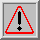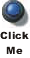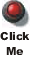# PHY138Y - Mechanics - Class 9 - Wed. Oct. 13, 2004

## Introduction

"Music is a hidden practice of the soul, which does not know that it is doing mathematics."

-- Leibniz

## Announcements

### Pre-Class Quizzes

#### #3

• Due last Wednesday
• Class average: 91.2%

#### #5

• Now released
• Due by 10 AM Wednesday October 20
• On Chapter 10
• This is the last Pre-Class Quiz of the Mechanics section of PHY138.

### MP Problem Sets

#### #3

• Due last Friday
• Question results:
• Work on a Sliding Block: 92% correct
• Pushing War: 99% correct
• Work-Energy Scaling: 96% correct
• Workhorses on the Erie Canal: 97% correct
• Holding Force of a Nail: 94% correct
• Power of One: 98% correct
• Power Dissipation Puts ...: 80% correct
• Time to complete: you = 85 minutes; the rest of the world = 70 minutes

I still don't understand why these assignments take you so much longer than the tens of thousands of mostly American first year Physics students who have done these questions over the past few years. Your overall performance in terms of percent correct, number of wrong answers, number of hints, etc. is virtually identical.

#### #5

• Now released
• Due by 5 PM Friday October 22
• On Chapter 8
• Other students have taken 38 minutes to complete the questions that are on this assignment. Maybe this one will take you somewhat less than an hour; that is the number we have been trying for all term.

### Next Representative Assembly

• Representatives from Wednesday tutorials
• This Friday, October 15, noon - 1 PM
• MP713

### Test #1

• Tuesday, November 2, 6 PM to 7:30 PMIf you have a conflict with this day and time contact Dr. Savaria in MP901A immediately.• It will cover all the material of this quarter of PHY138
• You may bring a single 8 1/2 by 11 inch sheet of paper on which you have written anything that you wish

We will be providing you with many more details about the test soon.

## Written Homework #5

 Due in the Drop Box for your tutorial group by Monday, October 18, by 5 PM.## Today's Class

Today we covered Chapter 7 Potential Energy of the textbook, but did not follow the textbook very closely. Here is an outline of how we discussed this material.

First, you should be aware that the whole concept of potential energy is in some sense just an abstraction of the idea of work. Although certainly a useful way to think about some mechanics problems, the concept is crucial for what comes in the third quarter of PHY138.

 We begin by considering the work done by gravity on an object of mass m that moves only under the influence of gravity. It begins at a height yi with some speed vi, and moves to a height yf where it has a speed vf. The total vertical distance it falls is h. The first realisation is that the work done by gravity is independent of the path, that it depends only on the initial vertical position and the final vertical position independent of how it got there. From the work-kinetic energy theorem we know that the quantity: m g y + 1/2 m v2 is the same value for all values of y.Forces whose work is independent of the path but depends only on the initial and final positions are called conservative. Another conservative force is the one exerted on a mass by a spring.

For the gravitational problem we define the potential energy U as equal to the quantity m g y. We call it the potential energy because it represents the potential for work to be done on the mass by gravity. Of course, as the work is actually done by gravity the potential for it to do work goes down, so the work actually done is the negative of the change in potential energy:

W = -U

We can say that the mechanical energy

Emech = U + K

is conserved for this situation.

Notice that in the above figure we did not specify the origin of the coordinate system, i.e. the point at which y = 0. We can, of course, choose the zero to be anywhere that we wish. For example:

• If we choose y = 0 to be at yf then yi = +h.
So the initial potential energy is m g h and the final potential energy is 0.
• If we choose y = 0 to be yi, then yf = -h.
So the initial potential energy is 0, and the final potential energy is - m g h.
• If we choose y = 0 to be half way between yi and yf then:
the initial potential energy is 1/2 m g h, and the final potential energy is - 1/2 m g h.

For all three of these choices, and in fact for any choice, the mechanical energy is still conserved.

Thus, we conclude that:

We can choose any position in space to be defined as having zero potential energy.

Of course, as usual with choices of a coordinate system, some choices of the zero of potential energy are mathematically easier than others.

Finally, there are 2 small matters to discuss:

1. Although it looks like the potential energy is just an abstract way of describing the work that can be done by a conservative force, it turns out that in some sense it is a real form of energy. For a spring, it is stored in the spring itself. For the gravitational system, it is stored in the gravitational field.
2. Non-conservative forces are those whose work depends on their path. Examples include friction and forces exerted in "sticky" collisions. For these forces, a potential energy can not be defined.

## In-Class Question

 We asked a question about the "pegged pendulum" and did a demonstration. Most of the class got the question correct: it rises to height B.## Another Demonstration

In class we also used an air track out of the laboratory for a demonstration. Some of you will be doing the experiment using this apparatus this term, and the apparatus will also be available next term. The Guide Sheet for the experiment is in your lab manual.